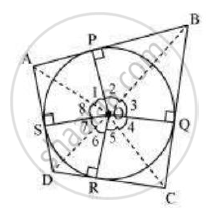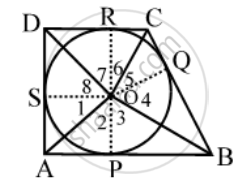Share

Books Shortlist

Prove that Opposite Sides of a Quadrilateral Circumscribing a Circle Subtend Supplementary Angles at the Centre of the Circle. - CBSE Class 10 - Mathematics

ConceptNumber of Tangents from a Point on a Circle

Question

Prove that opposite sides of a quadrilateral circumscribing a circle subtend supplementary angles at the centre of the circle.

Solution 1

Solution:Let ABCD be a quadrilateral circumscribing a circle centered at O such that it
touches the circle at point P, Q, R, S. Let us
join the vertices of the quadrilateral ABCD to the center of the circle.
Consider ΔOAP and ΔOAS,
AP = AS (Tangents from the same point)

OP = OS (Radii of the same circle)

OA = OA (Common side)

Thus, by Side-Side-Side criterion of congruence,

we have ΔOAP ≅ ΔOAS (SSS congruence criterion)

Therefore, A ↔ A, P ↔ S, O ↔ O

The corresponding parts of the congruent triangles are congruent. And thus, ∠POA = ∠AOS

∠1 = ∠8 Similarly,

∠2 = ∠3

∠4 = ∠5

∠6 = ∠7

∠1 + ∠2 + ∠3 + ∠4 + ∠5 + ∠6 + ∠7 + ∠8 = 360º

(∠1 + ∠8) + (∠2 + ∠3) + (∠4 + ∠5) + (∠6 + ∠7) = 360º

2∠1 + 2∠2 + 2∠5 + 2∠6 = 360º

2(∠1 + ∠2) + 2(∠5 + ∠6) = 360º

(∠1 + ∠2) + (∠5 + ∠6) = 180º

∠AOB + ∠COD = 180º

Similarly, we can prove that ∠BOC + ∠DOA = 180º

Hence, opposite sides of a quadrilateral circumscribing a circle subtend supplementary angles at the centre of the circle.

Solution 2

Given:  A quadrilateral ABCD circumscribes a circle with centre O.To Prove: ∠AOB+ ∠COD = 180° and ∠BOC + ∠AOD = 180°

Construction: Join OP, OQ, OR and OF

Proof: We know that the tangents drawn from an external point of a circle subtend equal angles at the centre.

∴ ∠1 = ∠ 2, ∠ 3 = ∠ 4, ∠ 5 = ∠ 6 and ∠7= ∠8

And, ∠1 + ∠2 + ∠3 + ∠4 + ∠5 + ∠6 + ∠7 + ∠8 = 360° [∠s at a point]

⇒ 2 (∠2 + ∠3) + 2 (∠6 + ∠7) = 360° and 2 (∠1+ ∠8) + 2(∠4 + ∠5) = 360°

⇒ ∠2 + ∠3 + ∠6 + ∠7 = 180° and ∠1 + ∠8 + ∠4 + ∠5 = 180°

⇒ ∠AOB + ∠COD = 180° and ∠AOD + ∠BOC = 180°

Is there an error in this question or solution?

Video TutorialsVIEW ALL 

Solution Prove that Opposite Sides of a Quadrilateral Circumscribing a Circle Subtend Supplementary Angles at the Centre of the Circle. Concept: Number of Tangents from a Point on a Circle.
S